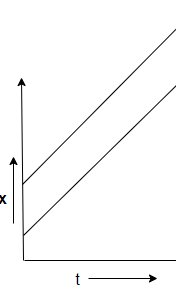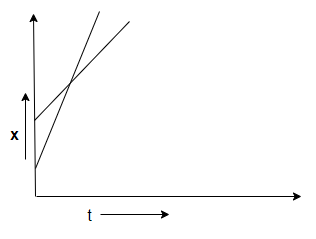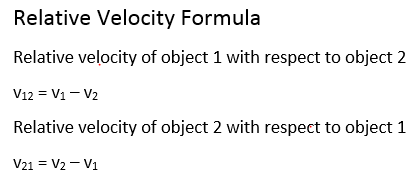# Relative velocity

## Relative velocity

• Consider two objects A and B moving with uniform velocities vAand vB along two straight and parallel tracks.
• Let xOAand xOB be their distances from origin at time t=0 and xAand xB be their distances from origin at time t.
• For object A
xA = xOA + vAt               (18)
and for object B
xB = xOB + vBt                (19)
subtracting equation 18 from 19
xB - xA = ( xOB - xOA ) + ( vB - vA) t                          (20)
• Above equation 20 tells that as seen from object A , object B seems to have velocity ( vB - vA) .
• Thus ( vB - vA ) is the velocity of object B relative to object A. Thus,
vBA = ( vB - vA )                (21)
• Similarly velocity of object A relative to object B is
vAB = ( vA - vB )                (22)
• If vB = vA then from equation 20
xB - xA = ( xOB - xOA )
i.e., two objects A and B stays apart at constant distance.• vA > vB then (vB - vA ) would be negative and the distance between two objects will go on decreasing by an amount ( vA - vB ) after each unit of time. After some time they will meet and then object A will overtake object B.• If vA and vB have opposite signs then magnitude of vBA or vAB would be greater then the magnitude of velocity of A or that of B and objects seems to move very fast.

## Relative velocity Conceptual questions

A.
A gun is mounted on a train roof .Train is travelling with the velocity v in north direction.A car is also moving on a parallel track with train with velocity w in north direction.Two bullet are fired from the muzzle of the gun . Take north as positive and south as negative
Question 1.
Bullet one is fired in the north direction with the muzzle velocity $u$ . Find the velocity of the bullet as seen from the observer on the earth
a. $u+v$
b. $u-v$
c. $u$
d. $v$

Question 2.
find the velocity of the bullet as seen from the observer on the moving car
a. $u+v-w$
b. $u-v-w$
c. $u$
d. $v$

Question 3
Second Bullet is fired in the south direction with the muzzle velocity $u$.Find the velocity of the bullet as seen from the observer on the earth
a. $u+v$
b. $v-u$
c. $u$
d. $v$

Question 4.
find the velocity of the second bullet as seen from the observer on the moving car
a. $u+v-w$
b. $v-u-w$
c. $u$
d. $v$

1. Velocity of bullet w.rt to train=u
Velocity of train = v
Velocity of bullet w.rt to train=Velocity of bullet w.r.t earth -Velocity of train w.r.t earth
So Velocity of bullet w.r.t earth =Velocity of bullet w.rt to train+Velocity of train w.r.t earth
$=u+v$
2. Velocity of bullet w.r.t earth =u +v
Velocity of Car w.r.t earth =w
Velocity of bullet w.rt to car=Velocity of bullet w.r.t earth -Velocity of car w.r.t earth
$=u+v-w$
3. Velocity of bullet w.rt to train= -u
Velocity of train = v
Velocity of bullet w.rt to train=Velocity of bullet w.r.t earth -Velocity of train w.r.t earth
So Velocity of bullet w.r.t earth =Velocity of bullet w.rt to train+Velocity of train w.r.t earth
$=-u+v= v-v$
4. Velocity of bullet w.rt to train=-u
Velocity of train w.r.t earth =v
velocity of car w.r.t earth  =w
Velocity of bullet w.rt to car=Velocity of bullet w.r.t earth -Velocity of car w.r.t earth
$= v-u-w$

B.
A train is moving in the west direction with a velocity 15m/s.A monkey runs on the roof of the train against its motion with a velocity 5m/s with respect to train .Take the motion along west as positive
Question 5.
Velocity of train relative to its driver
a. 0
b. 15 m/s
c. -15 m/s
d. 20 m/s

Question 6.
What is the velocity of train with respect to monkey
a. 5m/s
b -5 m/s
c. 15 m/s
d -15 m/s

Question 7.
find the velocity of monkey with respect to ground
a. 5 m/s
b. -5 m/s
c. 10 m/s
d. -10 m/s

5.
Velocity of train w.r.t to driver=Velocity of train w.r.t earth -Velocity of driver w.r.t earth
so =0
6.
Given
velocity of train = 15m/s
velocity of monkey w.r.t train=-5 m/s
Now velocity of monkey w.r.t train=-velocity of train w.r.t monkey
So velocity of train w.r.t monkey = 5 m/s
7.  velocity of train = 15m/s
velocity of monkey w.r.t train=-5 m/s
velocity of monkey w.r.t train = velocity of monkey with respect to ground   - velocity of train
-5 =velocity of monkey with respect to ground   - 15
velocity of monkey with respect to ground   =10 m/s

## Summary

Here is the summary for Relative Velocity in One dimension